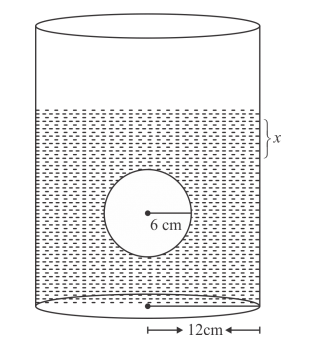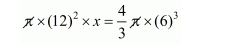# In a cylindrical vessel of diameter 24 cm,Question:

In a cylindrical vessel of diameter 24 cm, filled up with sufficient quantity of water, a solid spherical ball of radius 6 cm is completely immersed. Find the increase in height of water level.

Solution:

Radius of spherical ball r = 6 cm, radius of cylindrical vessel r1 = 12 cm

Since, the ball completely immersed into the vessel, the water level is increased.

Let the height of increased level.Therefore,

The volume of increase water level = volume of ball'

$144 x=\frac{4}{3} \times 216$

$144 x=4 \times 72$

$x=\frac{4 \times 72}{144}$

$x=2 \mathrm{~cm}$

Hence, the level of water increased by 2 cm.